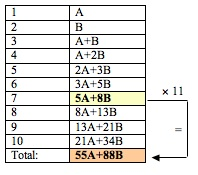# Fibonacci tricks from Palm Breeze CAFE

Posted by: Gary Ernest Davis on: November 17, 2010Here’s the algebra:x

x

x

x

x

x

x

More generally, if$F(n)$ is the$n^{th}$ Fibonacci number, where$F(1)=A, F(2)=B \textrm{ and } F(n+2)=F(n+1)+F(n)$, and$S(n)$ is the sum of the Fibonacci numbers$F(1)+\ldots+F(n)$ then$S(6+4k)$Â  is an integer multiple of$F(5+2k)$.

For example,$S(14)=F(1)+\ldots+F(14)=29\times F(9)$, and$S(98)=F(1)+\ldots+F(98)=17393796001\times F(51)$.

### 1 Response to "Fibonacci tricks from Palm Breeze CAFE"[…] Our thanks to the Republic of Maths blog that brought this to our attention, via this video link. […]